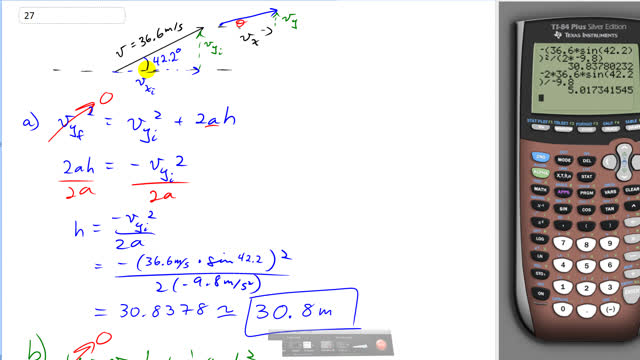## You are here

Question:

A projectile is fired with an initial speed of 36.6 m/s at an angle of $42.2^\circ$ above the horizontal on a long flat firing range. Determine

1. the maximum height reached by the projectile,
2. the total time in the air,
3. the total horizontal distance covered (that is, the range), and
4. the speed of the projectile 1.50 s after firing.

Giancoli, Douglas C., Physics: Principles with Applications, 7th Ed., ©2014. Reprinted by permission of Pearson Education Inc., New York.
The question will be visible after logging in, as required by Pearson Education Inc.

1. $30.8\textrm{ m}$
2. $5.02\textrm{ s}$
3. $136\textrm{ m}$
4. $28.9\textrm{ m/s}$

# Giancoli 7th Edition, Chapter 3, Problem 27

(5:12)